# Collinearity

Mason and Emma are playing near the bank of a river.

When Mason drops a few paper boats in the river, he observes that these paper boats were flowing in a straight line and behaving like points after traveling some distance.

These flowing boats which appear like points on a line are called collinear points.

But do you know what is collinearity?

Let's discuss collinearity!In this section, we will explore the meaning of collinearity, as well as collinear points.

Check-out the interactive simulation to know more about the lesson and try your hand at solving a few interesting practice questions on collinearity at the end of the page.

## Lesson Plan

 1 What Is the Meaning of Collinearity? 2 Thinking Out of the Box! 3 Solved Examples on Collinearity 4 Challenging Questions on Collinearity 5 Interactive Questions on Collinearity

## What Is the Meaning of Collinearity?

### Collinearity Definition

Collinearity is the property of a set of points where three or more than three points lie on a single line.

We can say that a set of points that lie on a single line are collinear.

The term collinearity has been used to indicate that the objects lie on a single line or in a row.

### Collinear Points Examples

Consider the following three points:

A(−3,−1), B(−1,0), and C(1,1)

If we plot these points in the cartesian plane, we find that they are collinear.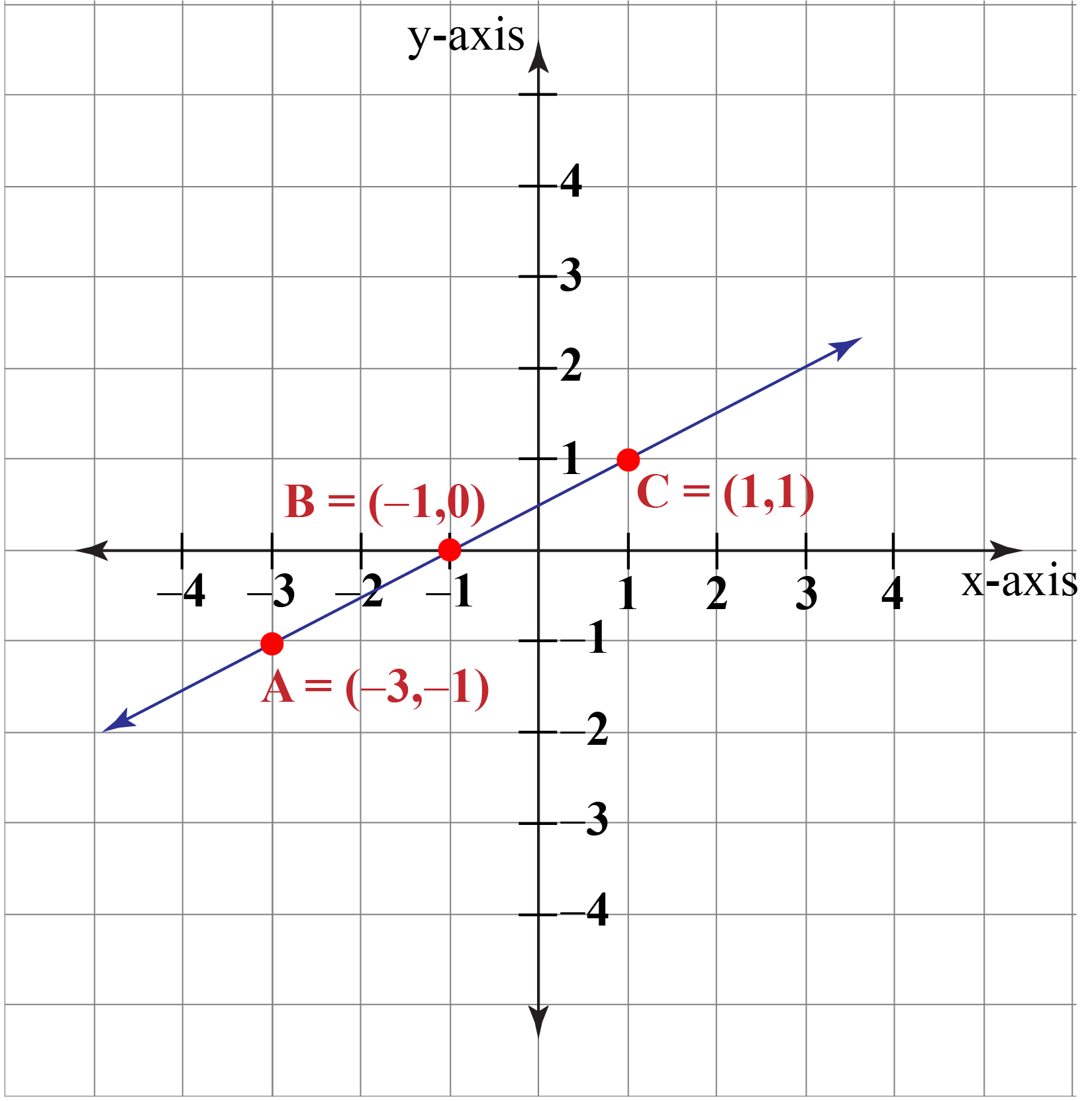Collinear Points and Non-Collinear Points.

If a line can pass through three or more points, the points are said to be collinear points.

In the given figure, points A, B, C, and D are collinear points.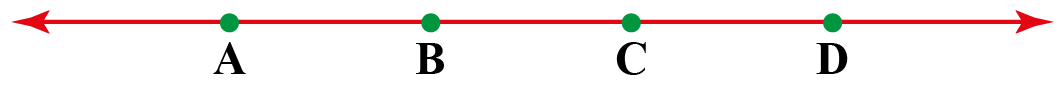If it is not possible to draw a straight line through three or more points, they are said to non-collinear points.

In the given figure, points I, G, and H are examples of non-collinear points.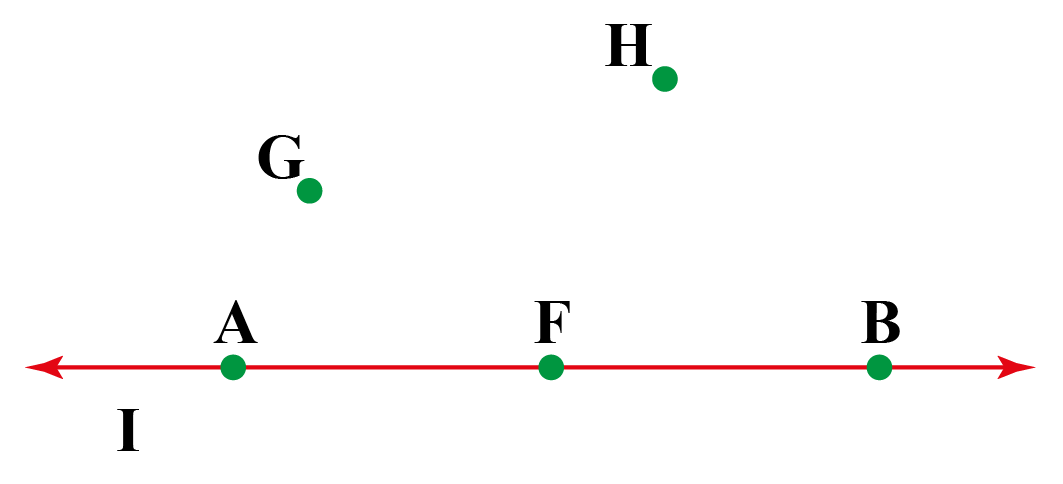## How To Determine If Points Are Collinear?

There are various methods to find out whether three points are collinear or not.

Each of the methods uses a collinear points formula to check whether the points are collinear or not.

### Using Distance Formula

Consider three points P, Q, and R.

Three points P, Q, R will be collinear if

Length of PQ + Length of QR = Length of PR

The formula for the distance $$d$$ between two points whose coordinates are $$(x_{1},y_{1})$$ and $$(x_{2},y_{2})$$ is:

 $$d = \sqrt{(x_{2}−x_{1})^2+(y_{2}−y_{1})^2}$$

This is called the Distance Formula.

For example:

Find whether the three points A(−3,−1), B(−1,0), and C(1,1) are collinear.

Three points will be collinear if AB + BC = CA

\begin{align*} \text{Length of AB} &= \sqrt{(x_{2}−x_{1})^2+(y_{2}−y_{1})^2} \\ &= \sqrt {(-1+3)^2+(0+1)^2} \\ &=\sqrt{5} \end{align*}

\begin{align*} \text{Length of BC} &= \sqrt{(x_{3}−x_{2})^2+(y_{3}−y_{2})^2} \\ &=\sqrt {(1+1)^2+(1-0)^2} \\ &=\sqrt{5} \end{align*}

\begin{align*} \text{Length of AC} &= \sqrt{(x_{3}−x_{1})^2+(y_{3}−y_{1})^2} \\ &=\sqrt {(1+3)^2+(1+1)^2} \\ &=\sqrt{20} = 2\sqrt 5 \end{align*}

### Slope Method

If three points $$P(x_{1},y_{1}), Q(x_{2},y_{2}), R(x_{3},y_{3})$$ are collinear, then the slope of the line formed by points $$P(x_{1},y_{1}), Q(x_{2},y_{2})$$ and $$Q(x_{2},y_{2}), R(x_{3},y_{3})$$ will be equal.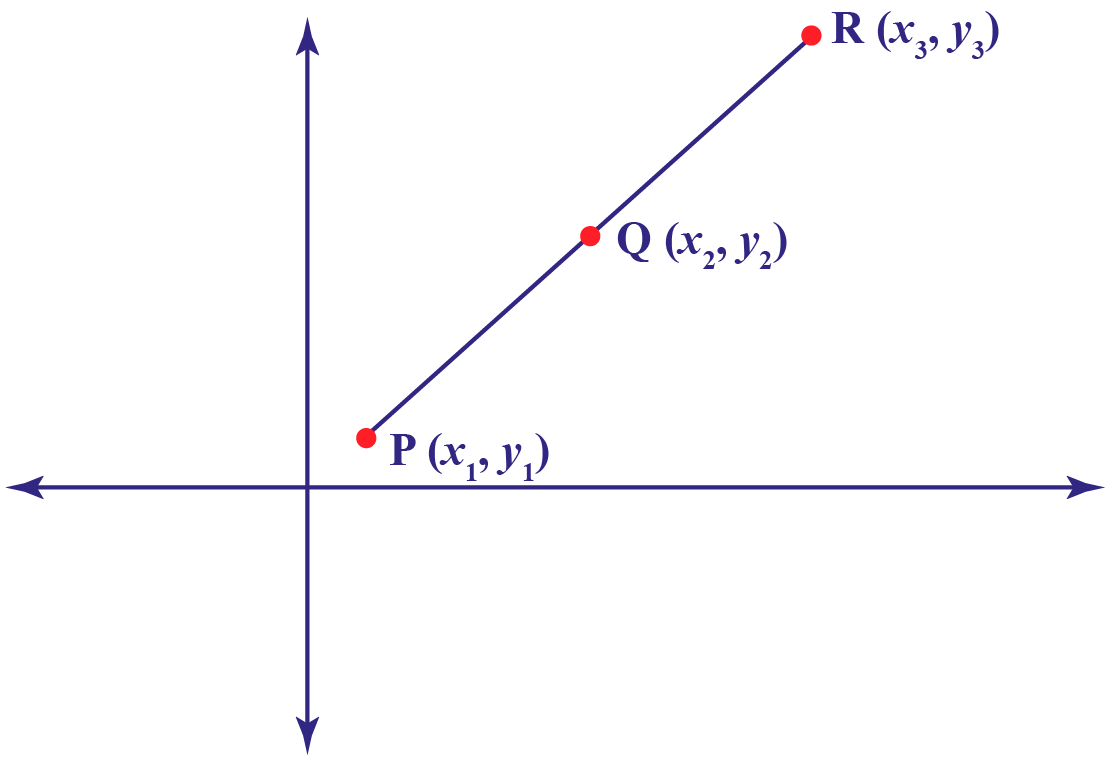The slope of line RQ is
$m_1=\dfrac{y_{3}−y_{2}}{x_{3}−x_{2}}$
The slope of line QP is
$m_2=\dfrac{y_{2}−y_{1}}{x_{2}−x_{1}}$
If three points are collinear, then
$m_1=m_2$
$\dfrac{y_{3}−y_{2}}{x_{3}−x_{2}}=\dfrac{y_{2}−y_{1}}{x_{2}−x_{1}}$

### Example

Check whether points P, Q, and R are collinear or not.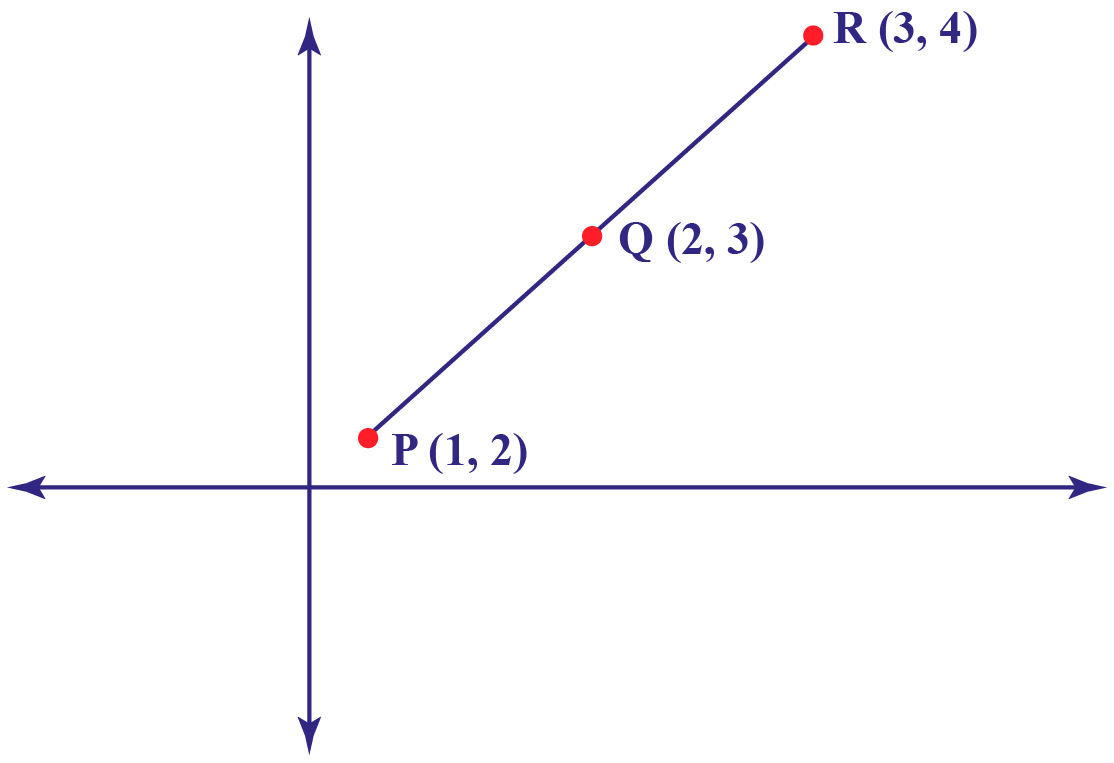### Solution

Slope of line RQ is

\begin{align*}m_1&=\dfrac{y_{3}−y_{2}}{x_{3}−x_{2}}\\m_1&=\dfrac{4−3}{3−2} \\m_1&=\dfrac{1}{1} \\ m_1&=1\end{align*}

Slope of line QP is

\begin{align*}m_2&=\dfrac{y_{2}−y_{1}}{x_{2}−x_{1}}\\m_2&=\dfrac{2-3}{1-2}\\m_2&=\dfrac{-1}{-1}\\m_2&=1\\m_1&=m_2\end{align*}

Thus, points P, Q, and R are collinear.

### Area of Triangle Method

If three points $$P(x_{1}, y_{1}), Q(x_{2},y_{2}), R(x_{3},y_{3})$$ are collinear, the area of the triangle formed by all three points $$P(x_{1},y_{1}), Q(x_{2},y_{2}), and R(x_{3},y_{3})$$ will be zero.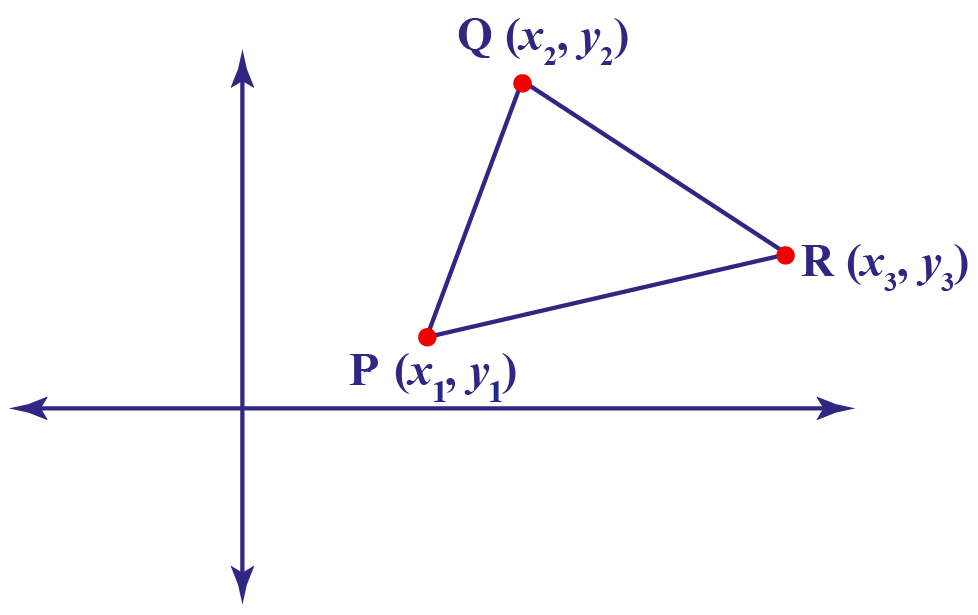If three points are collinear, the area of a triangle formed by the three points on a cartesian plane is zero.
$A = \dfrac{1}{2}|(x_1(y_2−y_3)+x_2(y_3−y_1)+x_3(y_1−y_2))| = 0$

Let's solve an example of collinearity.

### Example

Check whether the points $$(−1,−1), (1,1),$$ and $$(3,3)$$ are collinear or not.

### Solution

Let's take $$(−1,−1) \text{ as } (x_1,y_1), (1,1) \text{ as } (x_2,y_2), \text{ and } (3,3) \text{ as } (x_3,y_3)$$.

Substituting these value in the formula of area of the triangle:

\begin{align*}A&=\dfrac12|(x_1(y_2−y_3)+x_2(y_3−y_1)+x3(y_1−y_2))|=0 \\ A &= \dfrac12|(−1(1−3)+1(3−(−1))+3(−1(−1))|=0\\A&=\dfrac12|(2+4−6)|=0\\A&=\dfrac12|0|=0\\A&=0\end{align*}

Hence, the points are collinear.Think Tank
• Can three non-collinear points always form a triangle?
• Are two points always collinear?

## Solved Examples

 Example 1

Prove that the points P(-4, -2), Q(0, 2), and R(3, 5) are collinear points.

Solution

Let's prove that the points are collinear using the slope method.

If three points are collinear, they will satisfy the following condition:

$\dfrac {y_3−y_2}{x_3−x_2}=\dfrac{y_2−y_1}{x_2−x_1}$

Here,

$$(x_1, y_1)$$ is $$(-4, -2)$$

$$(x_2, y_2)$$ is $$(0, 2)$$

$$(x_3, y_3)$$ is $$(3, 5)$$

Put the values in the above equation,

$\dfrac {y_3−y_2}{x_3−x_2}=\dfrac{y_2−y_1}{x_2−x_1}$

$\dfrac {5−2}{3−0}=\dfrac{2−(-2)}{0−(-4)}$

$\dfrac {3}{3}=\dfrac{4}{4}$

$1=1$

L.H.S. = R.H.S.

Hence, the points are collinear.

 $$\therefore$$ Points P(-4, -2), Q(0, 2) and R(3, 5) are collinear.
 Example 2

Sam wants to find the value of $$k$$ for which the given points J(0, -2), K(2, 0), and L(5, $$k$$) are collinear.

Solution

Given: Points J, K, and L are collinear.

If three points are collinear, the area of the triangle formed by these points is zero.

Here,

$$(x_1, y_1)$$ is $$(0, -2)$$

$$(x_2, y_2)$$ is $$(2, 0)$$

$$(x_3, y_3)$$ is $$(5, k)$$

$\text{Area of triangle} = \dfrac12|(x_1(y_2−y_3)+x_2(y_3−y_1)+x_3(y_1−y_2)| = 0$

Substituting the values in the above equation, we get,

\begin{align*} \dfrac12|(0(0−k)+2(k-(-2))+5(−2−0)| &= 0 \\ \dfrac12|( 0+ 2k + 4 -10)| &= 0\\ \dfrac12|(2k−6)| &= 0\\ |(k-3)| &= 0\\ k &= 3 \end{align*}

 $$\therefore$$ k =3Challenging Questions
• If vector $$\vec{ \text a} = \hat{ \text i} + 2 \hat{ \text j}$$ and vector $$\vec{ \text b} = \text a \hat{i}−2 \text b \hat{j}$$ are collinear vectors with opposite directions, what is the value of a?

## Interactive Questions

Here are a few activities for you to practice. Select/Type your answer and click the "Check Answer" button to see the result.

## Let's Summarize

This mini-lesson targeted the fascinating concept of collinearity. The math journey around the collinearity starts with what a student already knows, and goes on to creatively crafting a fresh concept in the young minds. Done in a way that not only it is relatable and easy to grasp, but also will stay with them forever. Here lies the magic with Cuemath.

At Cuemath, our team of math experts is dedicated to making learning fun for our favorite readers, the students!

Through an interactive and engaging learning-teaching-learning approach, the teachers explore all angles of a topic.

Be it worksheets, online classes, doubt sessions, or any other form of relation, it’s the logical thinking and smart learning approach that we, at Cuemath, believe in.

4. How do you prove something is collinear?

5. Are three points always collinear?

6. What is the property of collinearity?

7. What are the examples of collinearity?

## 1. Are collinear points also coplanar?

Yes, collinear are points also coplanar.

## 2. Can three collinear points form a triangle?

Since collinear points are those points that lie in a single line, it is impossible to form a triangle using collinear points.

## 3. What are non-collinear points?

Non-collinear points are the points that do not lie on the same line.

## 4. What is an example of a collinear?

When three or more points lie on the same line these points are collinear with each other.

## 5. How do you prove Collinearity?

The slope formula method is used to find that points are collinear. If the slope of any two pairs of points is the same then these points show collinearity.

## 6. What is the property of collinear?

The property of collinear is that the three points A, B, and C should lie on the same straight line.

## 7. What are non-collinear points in geometry?

Non-collinear points in geometry are the points that do not lie on the same line.

Coordinate Geometry
Coordinate Geometry
Grade 10 | Questions Set 1
Coordinate Geometry
Grade 9 | Questions Set 1
Coordinate Geometry